Question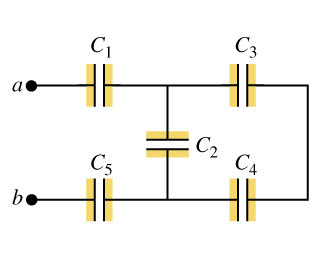In the figure (Figure 1) , C1 = C5 = 8.3 ?F and C2= C3= C4 = 4.2 ?F . The applied potential is Vab = 250 V .

A)Find Q1 Q2 Q3 Q4 Q5

B) Find V1 V2 V3 V4 V5

C3 and C4 are in series and their series combination is given as

C34 = C3 C4 /(C3 + C4) = (4.2 x 4.2)/(4.2 + 4.2) = 2.1 uF

C34 and C2 are in parallel and their parallel combination is given as

C234 = C2 + C34 = 4.2 + 2.1 = 6.3 uF

C1 , C234 and C5 are in series and their series combination is given as

1/C12345 = 1/C1 + 1/C234 + 1/C5

1/C12345 = 1/8.3 + 1/6.3 + 1/8.3

C12345 = 2.5 uF

Qtotal = C12345 Vab = 2.5 x 250 = 625 uC

Q1= Q5 = Qtotal = 625 uC              since C1 and C5 are in series with Vab

V1 = V5 = Q1/C1 = 625 / 8.3 = 75.3 volts

V2 = Vab - V1 - V5 = 250 - 75.3 - 75.3 = 99.4 volts

Q2 = C2 V2 = 4.2 x 99.4 = 417.5 uC

Q3 = Q4 = Qtotal - Q2 = 625 - 417.5 = 207.5 uC

#### Earn Coins

Coins can be redeemed for fabulous gifts.

Similar Homework Help Questions
• ### In the figure (Figure 1), C1 = C5 = 8.3 μF and C2= C3 = C4 = 4.3 μF. The applied potential is Vab = 250 V.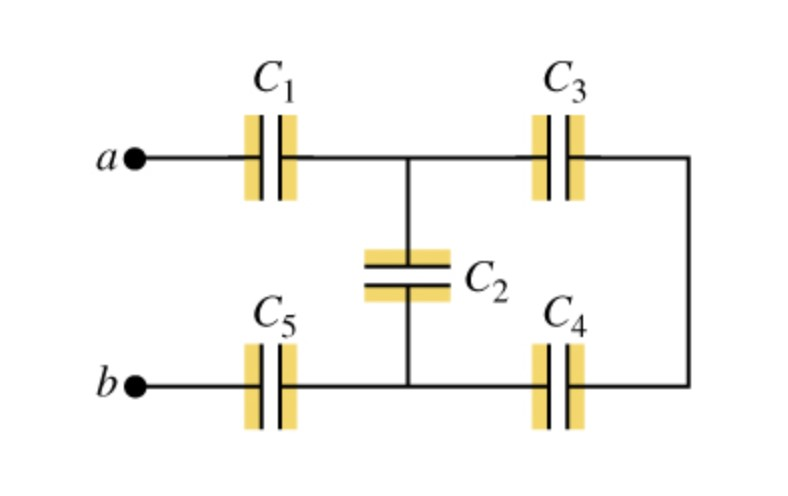In the figure (Figure 1), C1 = C5 = 8.3 μF and C2= C3 = C4 = 4.3 μF. The applied potential is Vab = 250 V.a) What is the equivalent capacitance of the network between points a and b?b) Calculate the charge on capacitor C1.c) Calculate the potential difference across capacitor C1.d) Calculate the charge on capacitor C2.e) Calculate the potential difference across capacitor C2.f) Calculate the charge on capacitor C3.g) Calculate the potential difference across capacitor C3.h) Calculate...

• ### In the figure (Figure 1) , C1 = C5 = 8.8 ?F and C2= C3 =...In the figure (Figure 1) , C1 = C5 = 8.8 ?F and C2= C3 = C4 = 4.2 ?F . The applied potential is Vab = 200 V . a. What is the equivalent capacitance of the network between points a and b? b. Calculate the charge on each capacitor and the potential difference across each capacitor

• ### In the figure (Figure 1), each capacitor has 4.70 uF and Vab = 29.0 V Figure...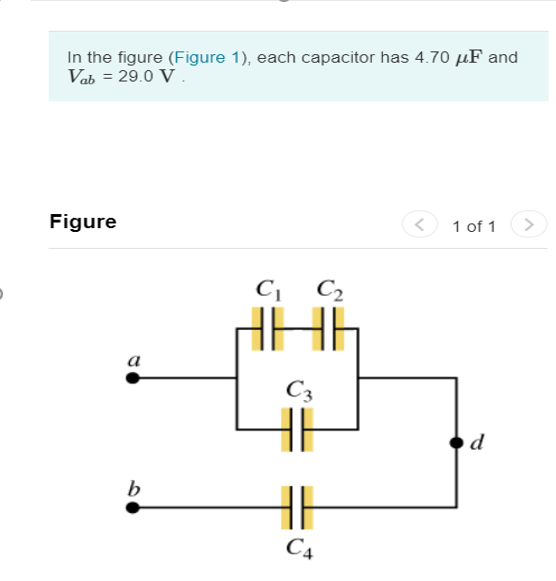In the figure (Figure 1), each capacitor has 4.70 uF and Vab = 29.0 V Figure < 1 of 1 > C C2 a C3 d b C4 (a) Calculate the charge on capacitor C1 Q1= (b) Calculate the potential difference across capacitor C1 V1= (c) Calculate the charge on capacitor C2 Q2= (d) Calculate the potential difference across capacitor C2 V2= (e) Calculate the charge on capacitor C3. Q3= (f) Calculate the potential difference across capacitor C3. V3= (g)...

• ### In the figure (Figure 1), each capacitor has 4.70 uF and Vab = 29.0 V Figure...In the figure (Figure 1), each capacitor has 4.70 uF and Vab = 29.0 V Figure < 1 of 1 > C C2 a C3 d b C4 (a) Calculate the charge on capacitor C1 Q1= (b) Calculate the potential difference across capacitor C1 V1= (c) Calculate the charge on capacitor C2 Q2= (d) Calculate the potential difference across capacitor C2 V2= (e) Calculate the charge on capacitor C3. Q3= (f) Calculate the potential difference across capacitor C3. V3= (g)...

• ### Calculate the Charge on C1, C2, C3, C4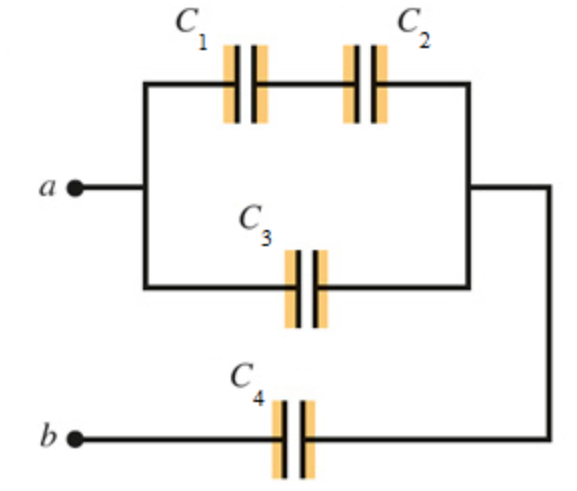Calculate the Charge on C1, C2, C3, C4Calculate the potential difference across C1, C2, C3, C4In (Figure 1), each capacitor has C 4.25 F and Vab 31.0V.

• ### In the figure a 23 V battery is connected across capacitors of capacitances C1 = C6 = 5.0 μF and C3 = C5=...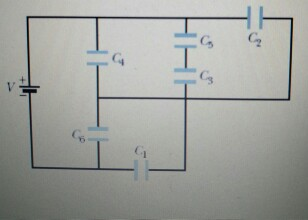In the figure a 23 V battery is connected across capacitors of capacitances C1 = C6 = 5.0 μF and C3 = C5= 2.0C2 = 2.0C4 = 5.0 μF. What are (a) the equivalent capacitance Ceq of the capacitors and (b) the charge stored by Ceq? What are (c) V1 and (d) q1 of capacitor 1,(e) V2 and (f) q2 of capacitor 2, and (g) V3 and (h) q3 of capacitor 3?

• ### Vbat = C4 C5 Consider the circuit shown above. The battery provides a voltage of Vbat...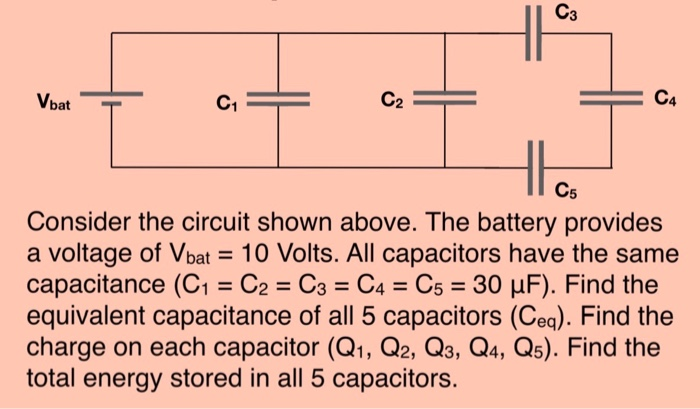Vbat = C4 C5 Consider the circuit shown above. The battery provides a voltage of Vbat = 10 Volts. All capacitors have the same capacitance (C1 = C2 = C3 = C4 = C5 = 30 uF). Find the equivalent capacitance of all 5 capacitors (Ceg). Find the charge on each capacitor (Q1, Q2, Q3, Q4, Q5). Find the total energy stored in all 5 capacitors.

• ### V1 C1 = 3 uF, C2 = 6 F, C3 = 4 uF, C4 = 2uF,...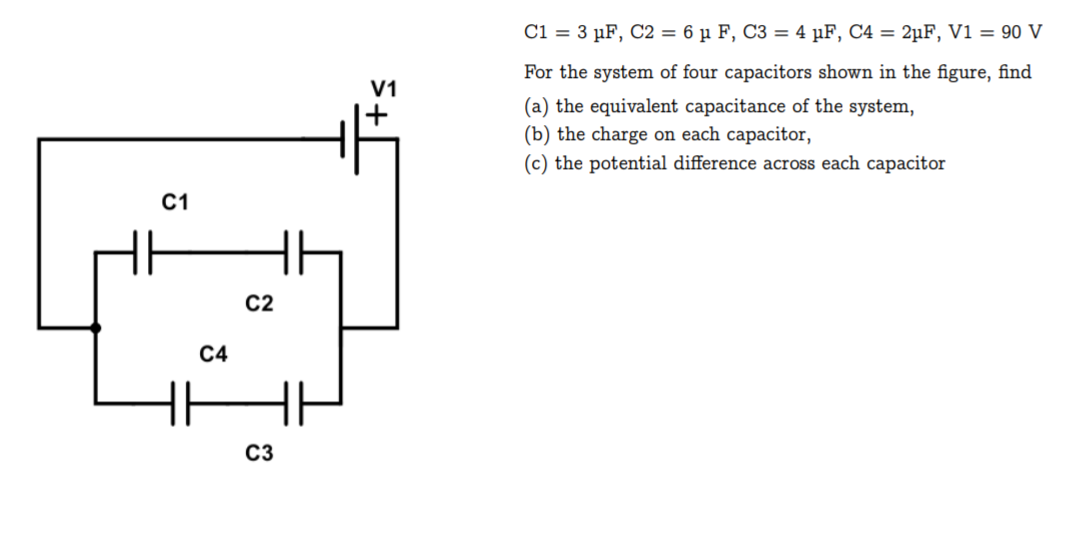V1 C1 = 3 uF, C2 = 6 F, C3 = 4 uF, C4 = 2uF, V1 = 90 V For the system of four capacitors shown in the figure, find (a) the equivalent capacitance of the system, (b) the charge on each capacitor, (c) the potential difference across each capacitor C1 C2 C4 C3

• ### Three capacitors with capacitances C1 = 6.3 ?F, C2 = 1.1 ?F, and C3 = 4.3...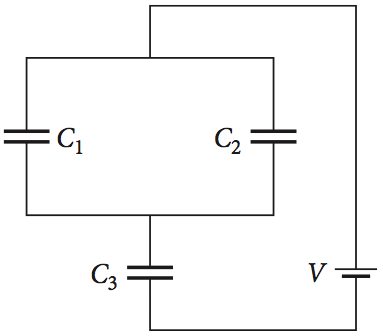Three capacitors with capacitances C1 = 6.3 ?F, C2 = 1.1 ?F, and C3 = 4.3 ?F are connected in a circuit as shown in the figure, with an applied potential of V. After the charges on the capacitors have reached their equilibrium values, the charge Q2 on the second capacitor is found to be 55. ?C. a) What is the charge, Q1, on capacitor C1? b) What is the charge, Q3, on capacitor C3? c) How much voltage, V,...

• ### They are all one question but many parts In (Figure 1), C1 = C5 = 9.0...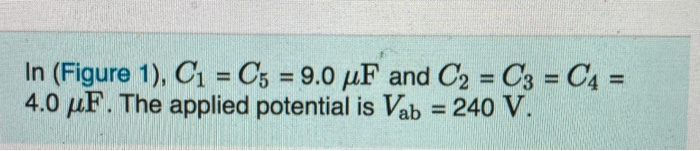They are all one question but many parts In (Figure 1), C1 = C5 = 9.0 uF and C2 = C3 = C4 = 4.0 pF. The applied potential is Vab = 240 V. In (Figure 1), C1 = Cs 9.0 uF and C2 = C3 = C4 = 4.0 F. The applied potential is Vob = 240 V. Part A What is the equivalent capacitance of the network between points a and b? Express your answer in farads. O...